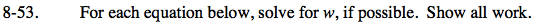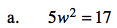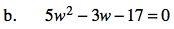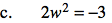Home > GC > Chapter 8 > Lesson 8.1.5 > Problem8-53

8-53.
1. For each equation below, solve for w, if possible. Show all work. Homework Help ✎

1. 5w2 = 17

2. 5w2 − 3w − 17 = 0

3. 2w2 = −3$\frac{5w^{2}}{5}= \frac{17}{5}$

$w^{2}= \frac{17}{5}$

$\sqrt{w^{2}}= \sqrt{\frac{17}{5}}$

w ≈ ± 1.84

Remember that since each equation has w² you should be looking for two possible answers.

It may help to use the Quadratic Formula.

w ≈ 2.17 and w ≈ 1.57No real solution because you can't take the square root of a negative number.